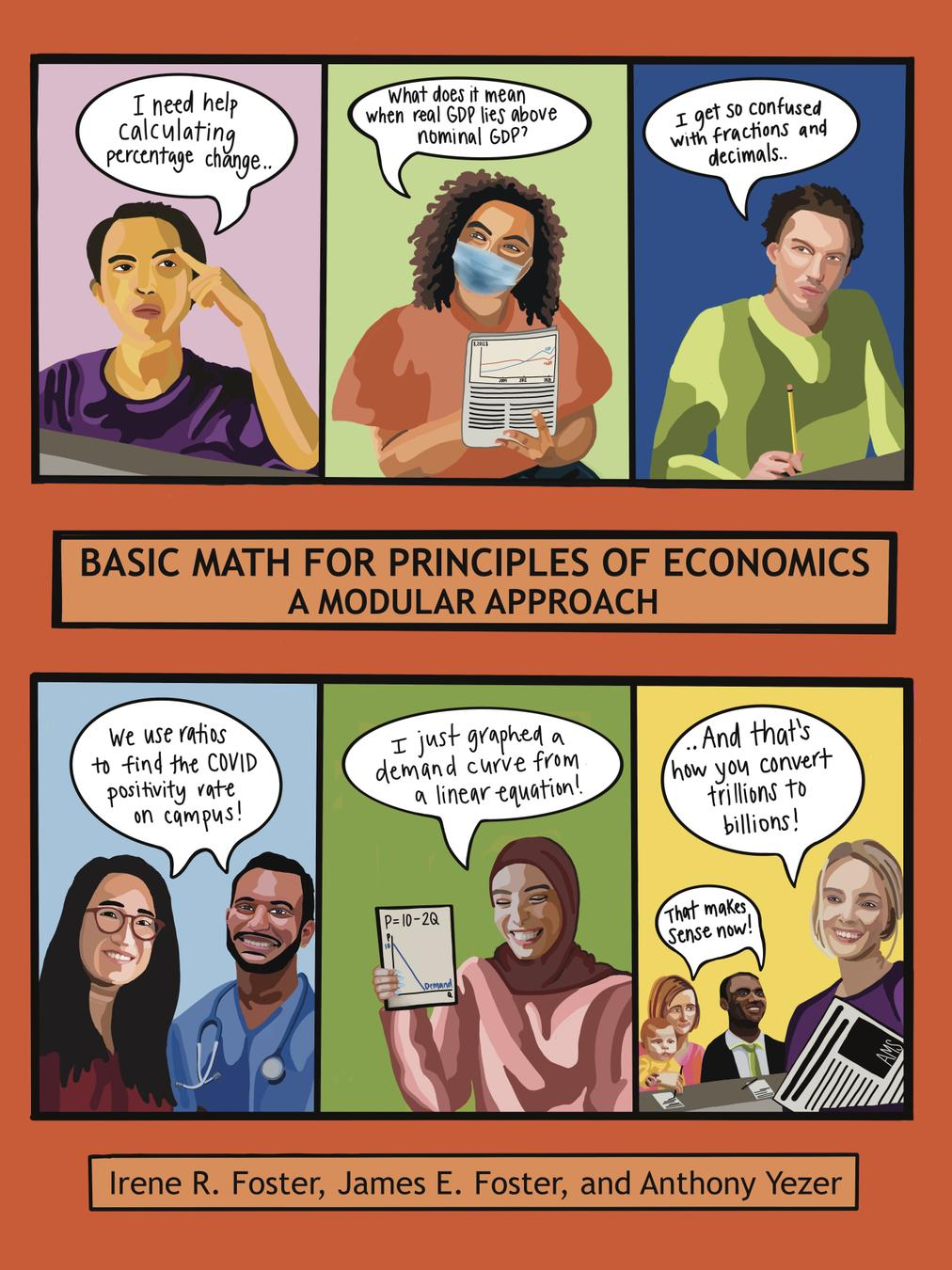# Basic Math for Principles of Economics: A Modular Approach

Irene Foster

Much of the material in this book was developed for Principles of Mathematics for Economics, an innovative new course at The George Washington University (GW) meant for students who don't feel prepared for a fast-paced introductory Microeconomics course their first semester of college. The course is meant for students weak in basic math, students not confident about their math skills, students not confident about taking economics, and students not having adequate study skills.Top Hat Interactive eText

requires a join code from instructor

\$43.2083%

of students say Top Hat helped them understand the course better.

Source: Top Hat Student Survey Spring 2023; n = 4,146

• Module 1 - An Introduction to Numbers
• Module 2 - Mastering Addition and Subtraction
• Module 3 - Mastering Multiplication and Division
• Module 4 - Understanding Data: The Mean, The Median and The Mode
• Module 5 - A Simple Introduction to Exponents
• Module 6 - What Exactly is a Fraction?
• Module 7 - More on Fractions
• Module 8 - Ratios, Rates, and Proportions - Are They Fractions?
• Module 9 - What Exactly is a Decimal?
• Module 10 - Basic Scientific Notation
• Module 11 - Fractions, Decimals, Percents and Percentages
• Module 12 - Percentage Change
• Module 13 - Algebraic Expressions
• Module 14 - Algebraic Equations